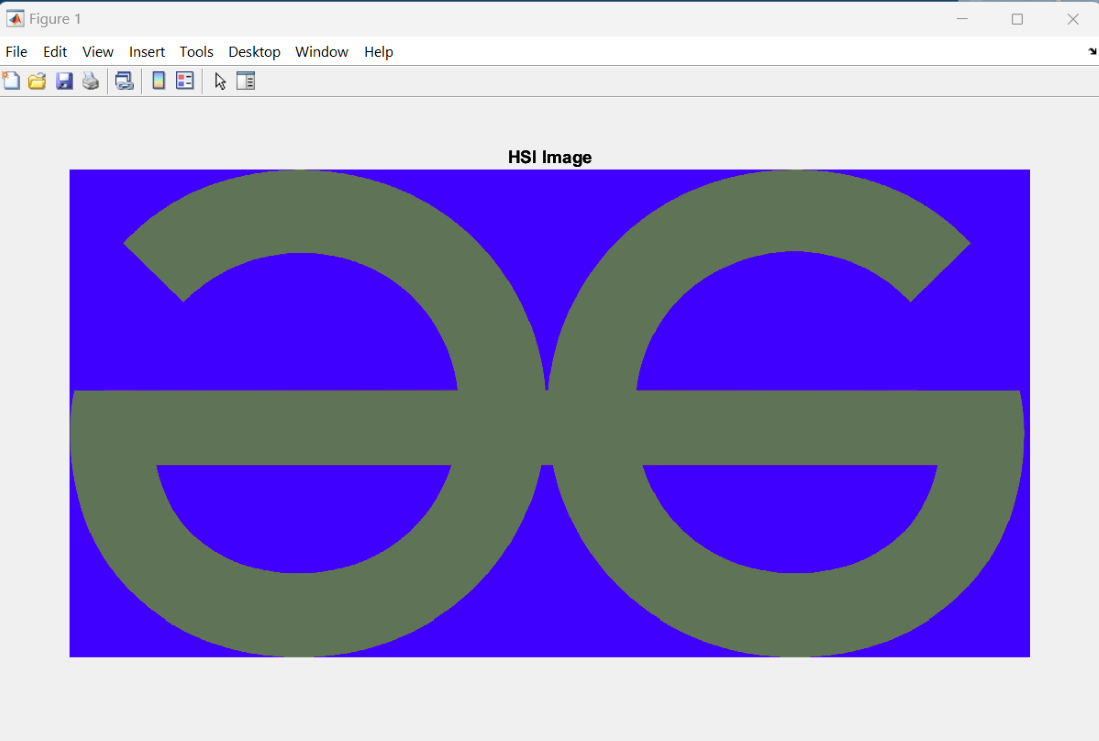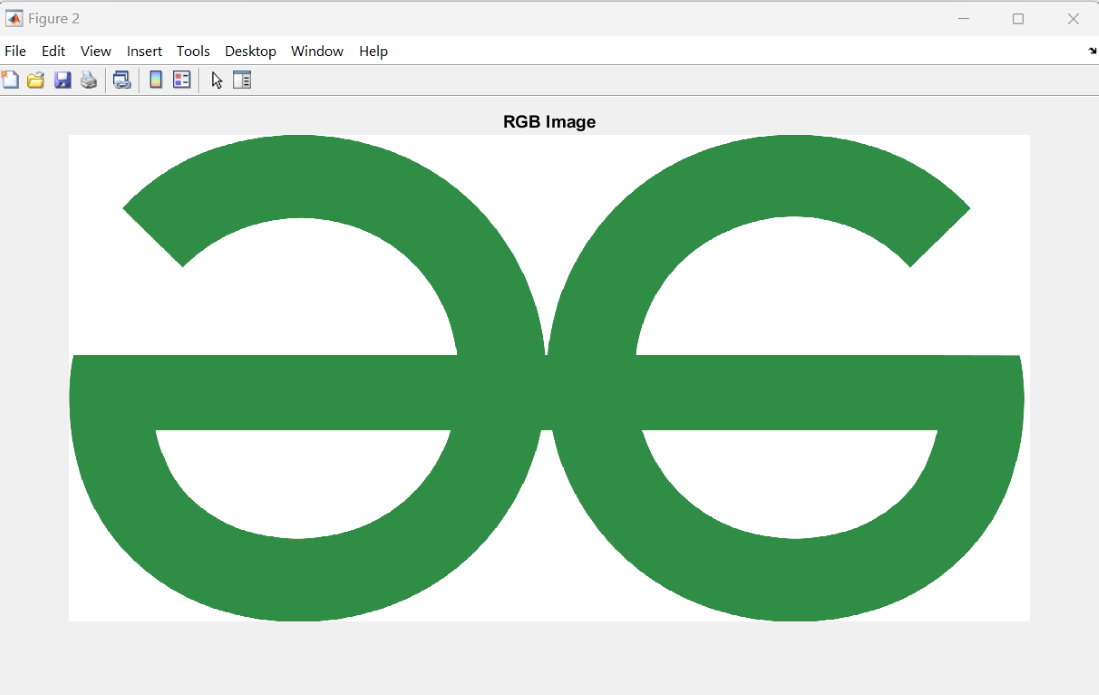Open in App
Not now

# How to Convert HSI Image to RGB Image in MATLAB?

• Difficulty Level : Medium
• Last Updated : 11 Jan, 2023

HSI stands for Hue Saturation Intensity. HSI is color space, it provides a way of numeric to readout the image that is corresponding to the color name contained. RGB it’s basically called the RGB triplet. in MATLAB the RGB image is a kind of  MxNx3 M cross N cross 3 arrays of colors pixel which is use three columns wide and each row contains three elements RGB (red, green, blue) triplet

In MATLAB image conversion is mostly performed operation in digital image processing. converting the color spaces of images the mostly used to analyze a subcategory or field of digital signal processing. MATLAB can not perform the direct HSI image conversion to RGB image, because MATLAB does not have any pre-built-in function for performing the HSI image conversion to RGB image. conversion of HSI to RGB image kind of matrix-based Mathematical equation problem.

So in this article, we will learn how to convert the HSI image to an RGB image in MATLAB following the line of code. First of all, you have to open your MATLAB editor and then create a new script for performing Conversion to RGB image color mode.

Example 1:

## Matlab

 `%  MATLAB program for conversion HSI to RGB image.``figure, ``% show the HSI image in figure1.``imshow(HSI);title(``'HSI Image'``); ` ` ``% Get the Hue, Saturation and Intensity components `` ``H1=HSI(:,:,1); `` ``S1=HSI(:,:,2); `` ``I1=HSI(:,:,3);` ` ``% Multiply h1 Hue with the range [0 360] `` ``H1=H1*360;                                              ``    ` ` ``% allocation of the r,g,b element.`` ``R1=zeros(size(H1));  ``% return an matrix values.`` ``G1=zeros(size(H1)); `` ``B1=zeros(size(H1)); `` ``RGB1=zeros([size(H1),3]); ``    ` ` ``%RG Sector(0<=H<120) `` ``B1(H1<120)=I1(H1<120).*(1-S1(H1<120)); `` ``R1(H1<120)=I1(H1<120).*(1+((S1(H1<120).*cosd(H1(H1<120)))`` ``./cosd(60-H1(H1<120)))); `` ``G1(H1<120)=3.*I1(H1<120)-(R1(H1<120)+B1(H1<120));    `` ``%Subtract 120 from H1 `` ``H2=H1-120; `` ` ` ``R1(H1>=120&H1<240)=I1(H1>=120&H1<240).`` ``*(1-S1(H1>=120&H1<240)); `` ``G1(H1>=120&H1<240)=I1(H1>=120&H1<240).`` ``*(1+((S1(H1>=120&H1<240).*cosd(H2(H1>`` ``=120&H1<240)))./cosd(60-H2(H1>=120&H1<240)))); `` ``B1(H1>=120&H1<240)=3.*I1(H1>=120&H1<240)`` ``-(R1(H1>=120&H1<240)+G1(H1>=120&H1<240)); `` ``%Subtract 240 from Hue `` ``H2=H1-240; ` ` ``G1(H1>=240&H1<=360)=I1(H1>=240&H1<=360)`` ``.*(1-S1(H1>=240&H1<=360)); `` ``B1(H1>=240&H1<=360)=I1(H1>=240&H1<=360)`` ``.*(1+((S1(H1>=240&H1<=360).*cosd(H2(H1>`` ``=240&H1<=360)))./cosd(60-H2(H1>=240&H1<=360)))); `` ``R1(H1>=240&H1<=360)=3.*I1(H1>=240&H1<=360)`` ``-(G1(H1>=240&H1<=360)+B1(H1>=240&H1<=360)); ` ` ``%Form RGB Image `` ``RGB1(:,:,1)=R1; `` ``RGB1(:,:,2)=G1; `` ``RGB1(:,:,3)=B1; `` ` ` ``% Present image in the range [0 255] `` ``RGB1=im2uint8(RGB1); `` ` ` ` ` ``figure, ``% show the image in the figure 2`` ``imshow(RGB1);title(``'RGB Image'``);`

Output 1:figure1 HSI image

Output 2:figure2 – RGB image

Explanation:

So in the above code firstly we show the HSI image in a figure which is in form of different colors means it is in Hue, Saturation Intensity values. then we obtain the HSI component, then multiply it with hue within the range 0 to 360. after that, we will allocate the RGB triplet with zeros.

After running the above code you will get two figures as output first is the HSI image as figure1, and then that HSI image will convert into an RGB image which shows in figure2.

My Personal Notes arrow_drop_up